# Using Python for Streaming Data with IteratorsUsing pandas read_csv iterator for streaming data

Next, the tutorial uses the Pandas read_csv() function and chunksize argument to read by chunk of the same dataset, World Bank World Development Indicator.

Another way to read data too large to store in memory in chunks is to read the file in as DataFrames of a certain length (using the chunksize).

First and foremost, import the pandas’ package, then use the .read_csv() function which creates an interable reader object. Then, it can use next() method on it to print the value. Refer below for the sample code.

```# Import the pandas package
import pandas as pd

# Initialize reader object: df_reader
df_reader = pd.read_csv('ind_pop.csv', chunksize=10)

# Print two chunks
print(next(df_reader))
print(next(df_reader))
```

The output of the above code which processes the data by chunk size of 10.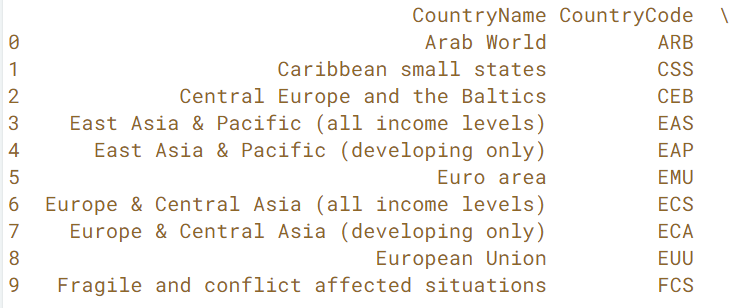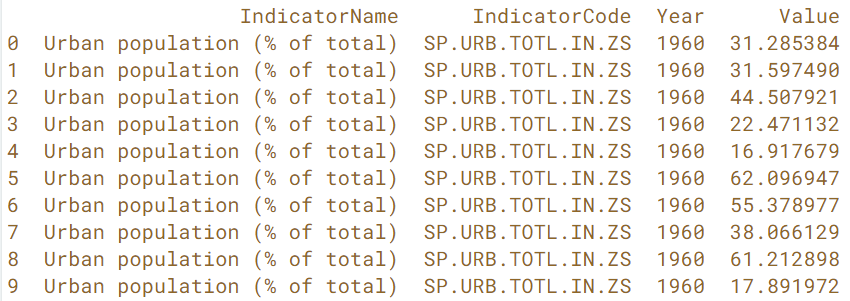The next 10 records will be from index 10 to 19.

Next, the tutorials require to create another DataFrame composed of only the rows from a specific country. Then, zip together two of the columns from the new DataFrame, ‘Total Population’ and ‘Urban population (% of total)’. Finally, create a list of tuples from the zip object, where each tuple is composed of a value from each of the two columns mentioned.

Sound a bit complicated now… Let see the sample code below:

```# Initialize reader object: urb_pop_reader
urb_pop_reader = pd.read_csv('ind_pop_data.csv', chunksize=1000)

# Get the first DataFrame chunk: df_urb_pop
df_urb_pop = next(urb_pop_reader)

# Check out the head of the DataFrame
print(df_urb_pop.head())

# Check out specific country: df_pop_ceb
df_pop_ceb = df_urb_pop.loc[df_urb_pop['CountryCode'] == 'CEB']

# Zip DataFrame columns of interest: pops
pops = zip(df_pop_ceb['Total Population'], df_pop_ceb['Urban population (% of total)'])

# Turn zip object into list: pops_list
pops_list = list(pops)

# Print pops_list
print(pops_list)
```

My output looks as below: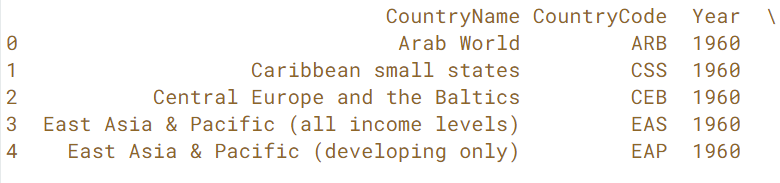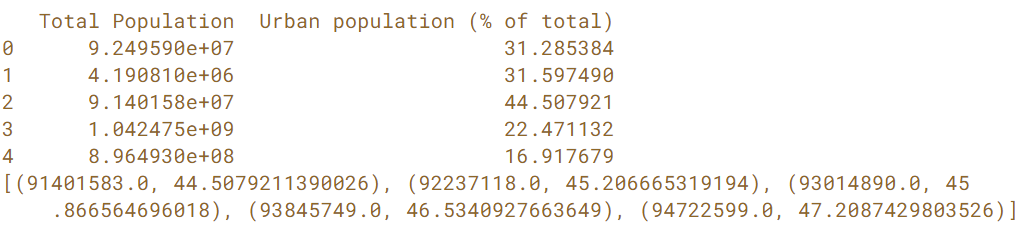Now, it requires to plot a scatter plot. The below source code contains the previous exercise’s code from the DataCamp itself. Therefore, there is a different of method used in this line,

```# Check out specific country: df_pop_ceb
df_pop_ceb = df_urb_pop.loc[df_urb_pop['CountryCode'] == 'CEB']

#From DataCamp
df_pop_ceb = df_urb_pop[df_urb_pop['CountryCode'] == 'CEB']
```

It requires to use list comprehension to create a new DataFrame. The values in this new DataFrame column is ‘Total Urban Population’, therefore, the product of the first and second element in each tuple.

Furthermore, because the 2nd element is a percentage, it needs to divide the entire result by 100, or alternatively, multiply it by 0.01.

Then, using the Matplotlib’s package, plot the scatter plot with new column ‘Total Urban Population’ and ‘Year’. It is quite a lot of stuff combined together up to this point. See the code and result of the plot shown below.

```# Code from previous exercise
urb_pop_reader = pd.read_csv('ind_pop_data.csv', chunksize=1000)
df_urb_pop = next(urb_pop_reader)
df_pop_ceb = df_urb_pop[df_urb_pop['CountryCode'] == 'CEB']
pops = zip(df_pop_ceb['Total Population'],
df_pop_ceb['Urban population (% of total)'])
pops_list = list(pops)

# Use list comprehension to create new DataFrame column 'Total Urban Population'
df_pop_ceb['Total Urban Population'] = [int(entry * entry * 0.01) for entry in pops_list]

# Plot urban population data
df_pop_ceb.plot(kind='scatter', x='Year', y='Total Urban Population')
plt.show()
```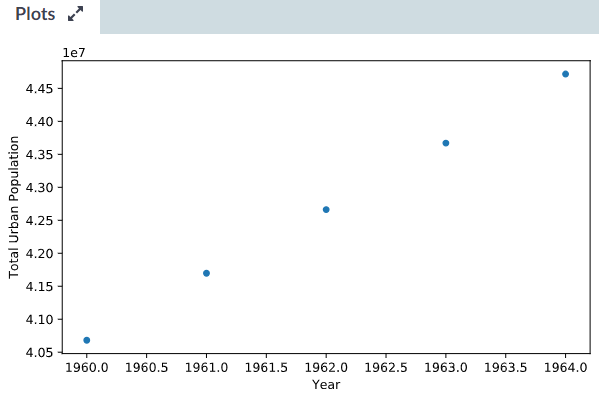I realized that the ‘Year’ is not in integer format. I printed out the value of the column ‘Year’ and it looked perfectly fine. Do you know what is wrong with my code above?

This time, you will aggregate the results over all the DataFrame chunks in the dataset. This basically means you will be processing the entire dataset now. This is neat because you’re going to be able to process the entire large dataset by just working on smaller pieces of it!

Below sample code consists of some of DataCamp’s code, so some variable names have changed to use their variable names. Here, it requires to append the DataFrame chunk into a variable called ‘data’ and plot the scatter plot.

```# Initialize reader object: urb_pop_reader
urb_pop_reader = pd.read_csv('ind_pop_data.csv', chunksize=1000)

# Initialize empty DataFrame: data
data = pd.DataFrame()

# Iterate over each DataFrame chunk
for df_urb_pop in urb_pop_reader:

# Check out specific country: df_pop_ceb
df_pop_ceb = df_urb_pop[df_urb_pop['CountryCode'] == 'CEB']

# Zip DataFrame columns of interest: pops
pops = zip(df_pop_ceb['Total Population'],
df_pop_ceb['Urban population (% of total)'])

# Turn zip object into list: pops_list
pops_list = list(pops)

# Use list comprehension to create new DataFrame column 'Total Urban Population'
df_pop_ceb['Total Urban Population'] = [int(tup * tup * 0.01) for tup in pops_list]

# Append DataFrame chunk to data: data
data = data.append(df_pop_ceb)

# Plot urban population data
data.plot(kind='scatter', x='Year', y='Total Urban Population')
plt.show()
```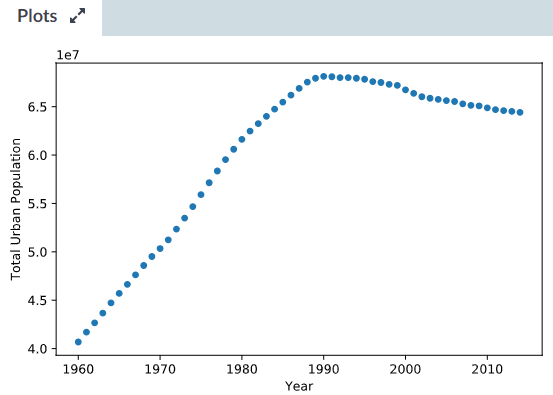I tried to compare the lines of codes used by DataCamp and mine.

```# Use list comprehension to create new DataFrame column 'Total Urban Population'
df_pop_ceb['Total Urban Population'] = [int(entry * entry * 0.01) for entry in pops_list]

# Plot urban population data
df_pop_ceb.plot(kind='scatter', x='Year', y='Total Urban Population')
plt.show()

# Use list comprehension to create new DataFrame column 'Total Urban Population'
df_pop_ceb['Total Urban Population'] = [int(tup * tup * 0.01) for tup in pops_list]

# Append DataFrame chunk to data: data
data = data.append(df_pop_ceb)

# Plot urban population data
data.plot(kind='scatter', x='Year', y='Total Urban Population')
plt.show()
```

Both of the df_pop_ceb look the same, so how come the scatter plots show differently. I still cannot figure out why my scatter plot is showing ‘Year’ with decimal point.

Lastly, wrap up the tutorial by creating an user defined function and taking two parameters, the filename and the country code to do all the above. The source code and another scatter plot is shown as below:

```# Define plot_pop()
def plot_pop(filename, country_code):

# Initialize reader object: urb_pop_reader
urb_pop_reader = pd.read_csv(filename, chunksize=1000)

# Initialize empty DataFrame: data
data = pd.DataFrame()

# Iterate over each DataFrame chunk
for df_urb_pop in urb_pop_reader:
# Check out specific country: df_pop_ceb
df_pop_ceb = df_urb_pop[df_urb_pop['CountryCode'] == country_code]

# Zip DataFrame columns of interest: pops
pops = zip(df_pop_ceb['Total Population'],
df_pop_ceb['Urban population (% of total)'])

# Turn zip object into list: pops_list
pops_list = list(pops)

# Use list comprehension to create new DataFrame column 'Total Urban Population'
df_pop_ceb['Total Urban Population'] = [int(tup * tup * 0.01) for tup in pops_list]

# Append DataFrame chunk to data: data
data = data.append(df_pop_ceb)

# Plot urban population data
data.plot(kind='scatter', x='Year', y='Total Urban Population')
plt.show()

# Set the filename: fn
fn = 'ind_pop_data.csv'

# Call plot_pop for country code 'CEB'
plot_pop('ind_pop_data.csv', 'CEB')

# Call plot_pop for country code 'ARB'
plot_pop('ind_pop_data.csv', 'ARB')
```

The scatter plot of the country code ‘ARB’ is shown below.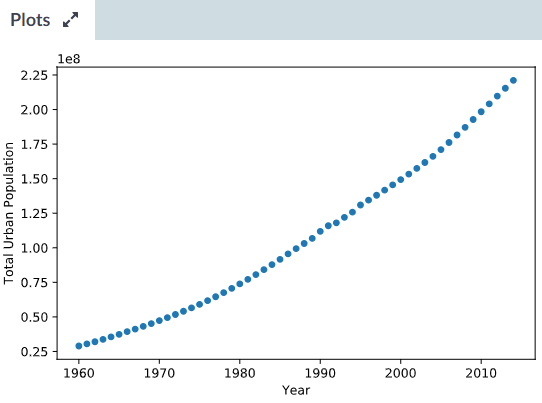Summary of the day:

• Use of user defined functions with parameters.
• Iterators and list comprehensions.
• Pandas’ DataFrame.
• Matplotlib scatter plot.
Advertisements